# Strings in VBA

String is the data type indicated to store text in VBA.

    Dim VarText As String 'Declaration of a variable of the data type String


To store text information in a String variable, the content must be enclosed in double quotation marks (" "):

    Dim VarText As String
VarText = "Some Name"


Although it is possible to store numeric information in text format, they will not behave in the same way as in numeric format. This will modify and limit their acceptable operators.

      Dim VarText As String
Dim VarNum As Single

VarText = "6" + "3"
MsgBox VarText 'This will result in "63" in text format

VarNum = 6 + 3
MsgBox VarNum 'This will result in 9

VarText = "6" / "3"
'This will generate an error: this operator does not exist for String

VarNum = 6 / 3
'This will make the division normally


The following are the main predefined functions for manipulating Strings in VBA.

## Key VBA Text Functions

### VBA UCase and LCase

The functions UCase and LCase make a string in uppercase and lowercase letters, respectively.

Function String Example Result
UCase Makes a string in uppercase letters
Sub UppercaseLetters()

Text = "Text Example"

MsgBox UCase(Text)

End Sub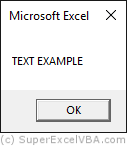TEXT EXAMPLE
LCase Makes a string in lowercase letters
Sub LowercaseLetters()

Text = "Text Example"

MsgBox LCase(Text)

End Sub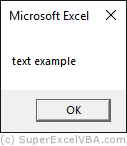text example

UCase and LCase are important functions for data standardization, since not all of your sources will be in the same case (uppercase or lowercase).

### VBA StrConv

Another function to change the text case is the StrConv. (which can work as UCase, LCase and can also make the first letters uppercase.

When using StrConv(string, conversion) it is necessary to include the desired conversion type as an argument.

Valor (conversion) Equivalent Description
StrConv(string,1) vbUpperCase Converts everything to uppercase
StrConv(string,2) vbLowerCase Converts everything to lowercase
StrConv(string,3) vbProperCase Converts the first letters to uppercase and the others for lowercase

Here is an example of StrConv using vbProperCase:

Function Argument Example Result
StrConv vbProperCase
Sub FirstUppercase()

Text = "text example"

MsgBox StrConv(Text, 3)
'StrConv(string, conversion)

End Sub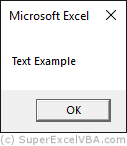Text Example

### VBA LTrim, RTrim and Trim

The LTrim, RTrim and Trim functions are used to remove spaces at both ends of a String:

Function String Example Result
LTrim Removes the spaces in the left end
Sub LeftEnd()

Text = "   Text Example   "

MsgBox ("Before using LTrim: " & Text & "..." _
& vbCrLf & vbCrLf & _
"After using LTrim: " & LTrim(Text) & "...")

End Sub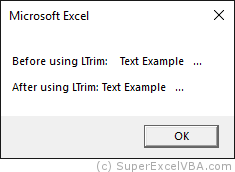Text Example ...
RTrim Removes the spaces in the right end
Sub RightEnd()

Text = "   Text Example   "

MsgBox ("Before using RTrim: " & Text & "..." _
& vbCrLf & vbCrLf & _
"After using RTrim: " & RTrim(Text) & "...")

End Sub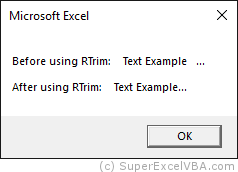Text Example...
Trim Removes the spaces in both ends
Sub Spaces()

Text = "   Text Example   "

MsgBox ("Before using Trim: " & Text & "..." _
& vbCrLf & vbCrLf & _
"After using Trim: " & Trim(Text) & "...")

End Sub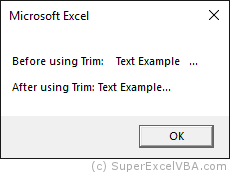Text Example...

The "..." was inserted after the variable to show the reduction of spaces.

vbCrLf is a function to add a line ("Enter") to the String.

### VBA Len

Len is a function that returns the total number of characters in a String.

Function String Example Result
Len Total characters
Sub Length()

Text = "Text Example"

MsgBox Len(Text)

End Sub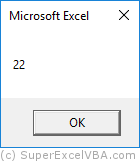12

All characters are counted, including whitespace characters.

Len can be used as a simple way to validate information.

Sub orderNumber
Dim onumber As String
onumber = Inputbox("What is your order number?")
If Len(onumber) = 10 Then
'All numbers must have 10 characters
MsgBox "We will process your request shortly."
Else
MsgBox "Invalid code. Please check the inserted number"
End If
End Sub


### VBA InStr and InStrRev

The InStr and InStrRev functions search within a String a given occurrence and return (counting from left to right in number of characters) the position it appears.

Function String Example Result
InStr Search the occurrence position from left to right
Sub WithinTheString()

Text = "text example"

MsgBox InStr(Text, "t")

End Sub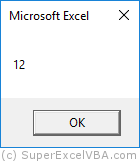12
InStrRev Search the occurrence position from right to left
Sub WithinTheStringReverse()

Text = "text example"

MsgBox InStrRev(Text, "t")

End Sub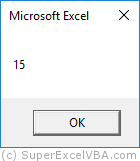15

These functions can be very useful when used together with Left and Right, as we will see later.

In both cases, InStr and InStrRev return:

• The position number, counting from the beginning of the String, that is, counting from left to right.
• The position of the first occurrence within the String,(InStr the left most, InStrRev the right most), starting from left to right.

If the returned value is 0, it means that the searched occurrence was not found within the String.

### VBA Left, Right and Mid

The Left, Right and Mid functions are used to extract parts of a line of text (String).

    MsgBox Left("Text example", 3) 'Retornará "Tex"
MsgBox Right("Text example", 3) 'Retornará "ple"
MsgBox Mid("Text example", 3, 4) 'Retornará "xt e"


Left, Right and Mid functions (such as Len, InStr and InStrRev) can be used in conjunction with other functions for better results.

Function String Example Result
Left Extracts characters to the left of a given position
Sub LeftCharacters()

Text = "Text Example Here"

WhiteSpace = InStr(Text, " ") 'Detects the position of the first whitespace character (from the left)
'WhiteSpace = 5

MsgBox Left(Text, WhiteSpace - 1)
'Left(string, [length])
'To extract the first word

End Sub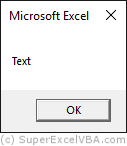Exemplo
Right Extracts characters to the right of a given position
Sub RightCharacters()

Text = "Text Example Here"

WhiteSpace = InStrRev(Text, " ") 'Detects the position of the first whitespace character (from the right)
'Whitespace = 13
WhiteSpace = Len(Text) - WhiteSpace
'To extract the last word
'WhiteSpace = 17 - 13

MsgBox Right(Text, WhiteSpace)
'Right(string, [length])

End Sub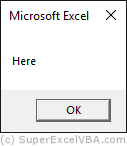Texto
Mid Extracts characters from within the string, between given positions
Sub CharactersMid()

Text = "Text Example Here"

Spc1 = InStr(Text, " ")
'Spc1 = 5

Spc2 = InStrRev(Text, " ")
'Spc2 = 13

Lngth = Spc2 - Spc1
MidText = Mid(Text, Spc1, Lngth)
'Mid(string, start, [length])

MsgBox Trim(MidText)

End Sub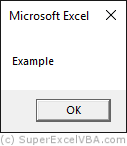de

In the arguments of the Mid function the initial position and the number of characters to be extracted must be indicated.

### VBA Replace

The Replace function is used to swap parts of the text with a desired one.

    Dim Text As String
Text = "Always, Always study VBA"

MsgBox Replace(Text, "Always", "Super")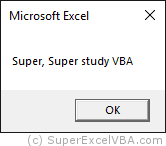### VBA String to numeric

It is possible to convert a data with type String to numeric with the help of functions:

Dim VarInt As Integer
VarInt = CInt("34") 'Converts "34" to the integer 34
MsgBox VarInt+3 'Returns 37

Function Definition
CByte Converts a String to type Byte
CInt Converts a String to type Integer
CLng Converts a String to type Long
CSng Converts a String to type Single
CDbl Converts a String to type Double
CCur Converts a String to type Currency
CDec Converts a String to type Decimal

You can convert a number to a String using CStr

VarText = CStr(33) 'Converts 33 to "33"
MsgBox VarText & "a" 'Returns "33a"